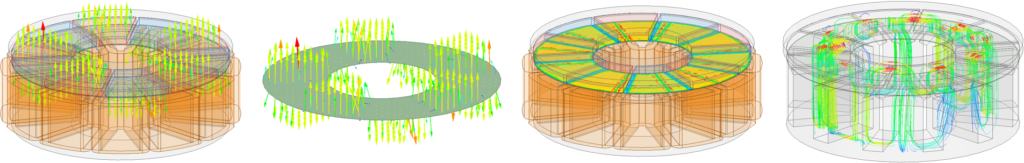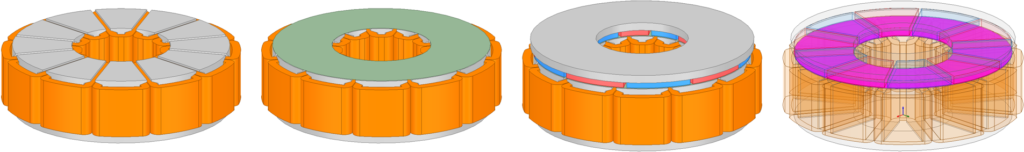In this course, we will follow the electromagnetic design of a case study motor:

• Axial-flux surface-mounted permanent magnet motor (sinusoidally excited)

In this example, we will consider the stator-rotor structure (considering the concentrated windings) and do analytic and finite element analysis. Please let me know if you have any questions via the following link:# Detailed course content:

1. Overview of course content
2. Discussing the case-study motors
3. Classification of design variables
4. Electromagnetic design procedure
5. Drawings of the motor structure
1. Torque-speed curve
2. Selection of base speed point
3. Calculation of electric torque
4. Calculation of voltages and currents
5. Derivation of the output equation
8. Calculation of the active volume
9. Calculation of the winding parameters
1. Creation of the stator
2. Creation of coils
3. Creation of Magnets
4. Creation of the rotor core
2. Calculation of flux distribution inside the active parts
3. Calculation of the flux linkages
4. Calculation of the reluctance factor
5. Calculation of the leakage factor
1. Reviewing HB-curve of magnets
2. What is intrinsic curve?
3. What is normal magnetization curve?
1. Setup the windings and excitations
2. Calculation of stator field
3. Calculation of direct and quadrature inductances (Ld and Lq)
4. Calculation of the static torque curve
1. Calculation of the motor BEMF
2. Calculating of cogging torque
4. Calculation of motor efficiency
1. Calculation of map curves
2. Calculation of torque-speed curve
3. Calculation of efficiency map
1. Discussing design objectives
2. Creation of 2D model for faster simulations
3. Optimal design of the motor (considering high-power density objective)
1. Reviewing the course content
2. Q&A section

1.